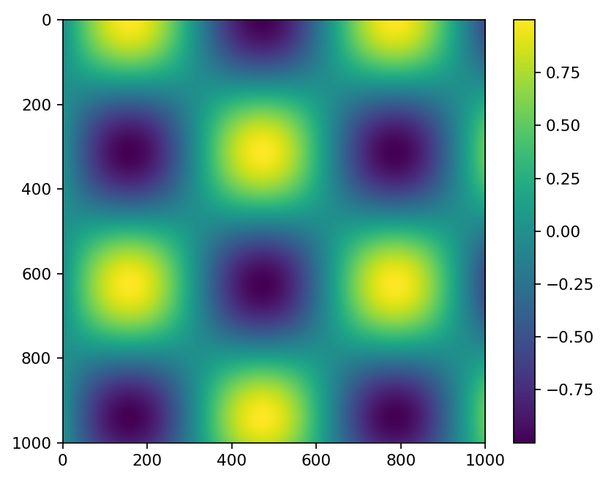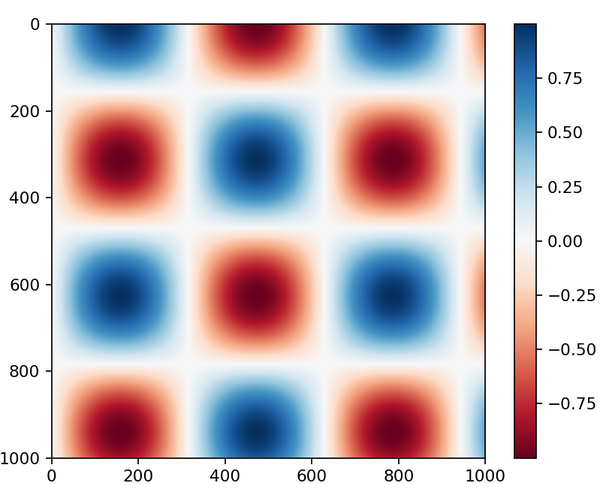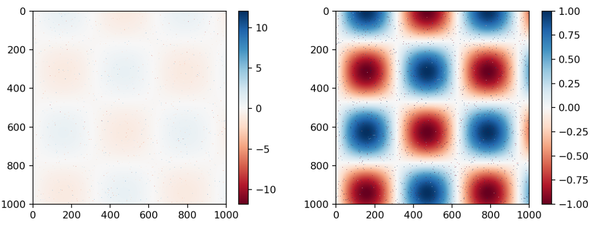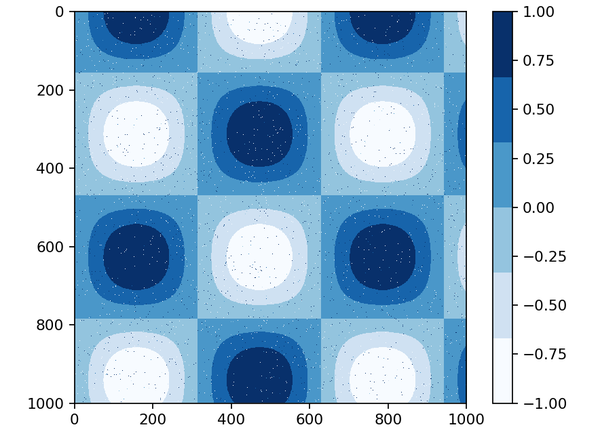# 颜色条

```%matplotlib notebook
import numpy as np
import matplotlib.pyplot as plt

x = np.linspace(0,10,1000)
I = np.sin(x)* np.cos(x[:,np.newaxis])
I.shape

plt.imshow(I)
plt.colorbar()
```• 顺序配色：由一组连续的颜色构成，比如binary和viridis
• 互逆色：通常由互补的颜色构成，比如RdBu或者PuOr
• 定性配色：随机顺序的一组颜色，比如rainbow或jet

jet是Matplotlib2.0之前的默认配色方案，已经比较老旧了。viridis是目前的默认配色方案。下面我们看看'RdBu'的配色：

```plt.imshow(I, cmap='RdBu')
plt.colorbar()
```colorbar可以看作是plt.Axes的一个实例，因此前面关于坐标轴的格式配置技巧都可以使用，下面我们看一个噪点图像，注意其中的clim方法：

```speckles = np.random.random(I.shape) < 0.01  # 制造bool噪点
I[speckles] = np.random.normal(0,3,np.count_nonzero(speckles)) # 生成噪点

plt.figure(figsize=(10,3.5))
plt.subplot(1,2,1)  # 多子图模式
plt.imshow(I, cmap='RdBu')
plt.colorbar()

plt.subplot(1,2,2)
plt.imshow(I, cmap='RdBu')
plt.colorbar(extend='both') # 颜色条变成两端尖
plt.clim(-1,1)  # 限制颜色显示范围
``````plt.imshow(I, cmap=plt.cm.get_cmap('Blues',6))
plt.colorbar()
plt.clim(-1,1)
```